# Interpreting QA output in Excel

## Excel Help for Interpreting Qa Output In Excel in Excel Multiple Regression Analysis and Forecasting Template

### Interpreting Qa Output In Excel

Rate this:
(3/5 from 1 vote)Ok, I have a set of data in Excel and I then ran a multiple regression (also in Excel) but I don't know how to interpret the analysis of the statistical results. e.g The null hypothesis as it related to coefficients etc. Furthermore I have to make an economic or business analysis of the result. I don't know if this is the right place to ask for help (apologies if it isn't. I'm very sorry) but if there is anyone willing to help, I will gladly post the excel sheet (with data and regression result) here.Thanks. HappyPpl. Posted by HappyPpl on 20 Aug 2010
 Replies - Displaying 1 to 8 of 8 Order Replies By: Most Recent | Chronological | Highest Rated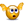Rate this: (3/5 from 1 vote) To interpret the multiple regression results, you should first look at the R squared which represents the percentage that changes in the independent variables (coefficients) effect the dependent variable (null hypothesis). Next, you you check at what level of confidence the result is statistically significant at using the F statistic. These are the basic tests; however more include multi-colinearity and auto-correlation. Multiple regression analysis assumes that data is numerical in nature (quantitative). If your input data is qualitative (such as Questions and Answers which I have interpreted 'QA output' as) then it is necessary to convert the data into numerical equivalents that can then be used as predictive components within the regression equation. For example, if the the answer can be dissatisfied, neutral or satisfied then this can be converted to a scale of 0-2 to represent the level of satisfaction. Excel Business Forums Administrator Posted by Excel Helper on 20 Aug 2010Rate this: (3/5 from 2 votes) Thanks for your reply. Actually by QA I meant Quantitative Analysis. Sorry about the confusion.I was able to do the regression in Excel but I'm truly at sea as to how to properly interpret this. I used Price (\$) as my dependent (Explained variable) Y axisthen the rest as independent (Explanatory variables) X axis.Is this also correct? Thanks. ﻿Can you help take a look at this and interpret using the questions below?Regression outputQuestions:A) Perform a multiple regression to explain the price of houses (US dollars) using whatever variables you wish from the data provided. -Based on this regression, prepare a report containing the following sections: 1. An analysis of the statistical results. You should cover such questions as the null hypothesis as it relates to coefficients, the sign (+/–) of coefficients, the meaning of the estimated coefficients as well as the overall predictive ability of the regression. 2. An economic or business analysis of the results. What do you conclude in general from the regression? Posted by HappyPpl on 20 Aug 2010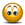Rate this:
(3/5 from 1 vote)
^^^Again, sorry I posted the regression output an an image but it never showed up.
Here again as a word document and I hope it does make sense.

 SUMMARY OUTPUT Regression Statistics Multiple R 0.950600603 R Square 0.903641506 Adjusted R Square 0.901788458 Standard Error 19828.391 Observations 319 ANOVA df SS MS F Significance F Regression 6 1.15037E+12 1.91728E+11 487.6514414 3.1114E-155 Residual 312 1.22668E+11 393165089.6 Total 318 1.27303E+12 Coefficients Standard Error t Stat P-value Lower 95% Upper 95% Intercept -6817.339436 7273.961335 -0.937225141 0.349367668 -21129.5598 7494.880931 Sq. Feet 63.33335967 2.912313097 21.74675509 1.81495E-64 57.60310281 69.06361652 Age -333.836386 94.88326877 -3.518390442 0.000498725 -520.528367 -147.1444049 Bedrooms -8444.831058 2176.762127 -3.879537848 0.000127688 -12727.82045 -4161.841664 Bathrooms -949.1948635 1176.548696 -0.806762072 0.42041812 -3264.167878 1365.778151 Garage # 26246.43488 2075.752197 12.64430067 7.20288E-30 22162.19227 30330.6775 Area 62040.98324 3684.608474 16.83787672 1.04501E-45 54791.16076 69290.80573
Posted by on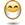Rate this: (3/5 from 2 votes) The multiple regression analysis completed with the analysis toolpak for Excel shows a 95% R squared that has a low confidence level of significance as shown by the F statistic.The set up is correct to predict house prices based on the independent variables. The null hypothesis requires a confidence level to be set (usually 95% at 2 standard deviations) to test whether or not (null hypothesis) that house price can be explained by the external factors at that level of confidence.The overall predictive ability is the R-squared. The coefficients of each independent variable (+ or -) represent the relationships e.g. as Age increases, price decreases by a factor of 333.While the analysis toolpak performs the raw regression analysis, it does not provide very useful descriptive information in order to interpret the results and validity of them. I suggest that you download and use our template from here which can be used fully for 30 days to conduct your analysis and provide advanced descriptive information as well as the ability to forecast for economic or business analysis. Excel Business Forums Administrator Posted by Excel Helper on 20 Aug 2010Rate this: (3/5 from 1 vote) Thanks again for your help.I have used the template you mentioned but I got these two errorsRuntime Error "6" overflow and Error "13".What do these errors indicate and how can they be resolved?Thanks. Posted by HappyPpl on 20 Aug 2010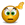Rate this: (3/5 from 1 vote) The run-time error 13 is due to incompatible data formats and usually arises from non numeric data such as text content within the input data.  Please verify that there are no strange characters within the titles or non numeric data within the observations.The overflow error is likely to be due to the previous error but may be due to a problem in calculating the number of observations or variables.  You can send through your template as an attachment as a reply to the notification email of this post so that we can investigate, resolve and post the solution here for you. Excel Business Forums Administrator Posted by Excel Helper on 21 Aug 2010Rate this: (3.5/5 from 2 votes) Hi and thanks for your reply once again.  I have sent the data/template as an attachment to as a reply to the e-mail notification as requested.  Could you kindly help check and resolve?  Thanks.  HappyPpl. Posted by HappyPpl on 21 Aug 2010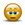Rate this: (3/5 from 1 vote) Thank you for sending through your data. The problem is with the Area independent variable data. You will notice that the regression analysis does actually complete and the error then appears when setting up the forecasting options. This is because the data for area has only 1 or 0 which is presumably for a Boolean result (either in the area or not). Normally this is not an issue, however the recurrence of only 1s followed by only 0s causes and issue with the algorithm to find linear, polynomial and exponential trends within the data. The solution is to reorder the observations slightly (such as putting the last row of dat as the first one) to create variability within the series. As a quick test, simply change cell G4 (first Area observation) of the Input sheet from 1 to 0 and re run the analysis. Excel Business Forums Administrator Posted by Excel Helper on 21 Aug 2010
 Displaying page 1 of 1

 Find relevant Excel templates and add-ins for Interpreting QA output in Excel in the Excel Business Solutions Directory